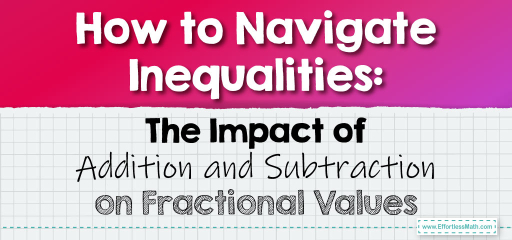# How to Navigate Inequalities: The Impact of Addition and Subtraction on Fractional Values

Inequalities are mathematical expressions that describe the relationship between two quantities. When working with fractions, understanding how addition or subtraction affects inequalities is crucial.In this guide, we’ll explore the nuances of inequalities when adding or subtracting fractions, ensuring you have a clear grasp of the concept.

## Step-by-step Guide to Navigate Inequalities:

1. Basics of Inequalities:

Inequalities use symbols like $$<$$ (less than), $$>$$ (greater than), $$\leq$$ (less than or equal to), and $$\geq$$ (greater than or equal to) to compare two values.

2. Understanding the Impact of Addition:

When you add the same value (fraction or whole number) to both sides of an inequality, the relationship between the two sides remains unchanged.

Example: If $$a > b$$, then $$a + c > b + c$$ for any real number $$c$$.

3. Understanding the Impact of Subtraction:

Similar to addition, when you subtract the same value from both sides of an inequality, the relationship remains unchanged.

Example: If $$a < b$$, then $$a – c < b – c$$ for any real number $$c$$.

4. Special Consideration for Negative Values:

When multiplying or dividing both sides of an inequality by a negative value, the direction of the inequality reverses.

Example: If $$a > b$$ and $$c < 0$$, then $$a \times c < b \times c$$.

5. Applying to Fractions:

When adding or subtracting fractions in inequalities, ensure the denominators are the same, and then compare the numerators. Remember, a larger numerator (with the same denominator) means a larger fraction.

### Example 1:

Given $$\frac{2}{5} < \frac{3}{5}$$, what happens when we add $$\frac{1}{5}$$ to both sides?

Solution:

Adding $$\frac{1}{5}$$ to both sides:

$\frac{2}{5} + \frac{1}{5} < \frac{3}{5} + \frac{1}{5}$

$\frac{3}{5} < \frac{4}{5}$

The Absolute Best Book for 5th Grade Students

### Example 2:

Given $$\frac{4}{7} > \frac{2}{7}$$, what is the result when we subtract $$\frac{1}{7}$$ from both sides?

Solution:

Subtracting $$\frac{1}{7}$$ from both sides:

$\frac{4}{7} – \frac{1}{7} > \frac{2}{7} – \frac{1}{7}$

$\frac{3}{7} > \frac{1}{7}$

### Practice Questions:

1. If $$\frac{3}{8} > \frac{1}{8}$$, what is the inequality after adding $$\frac{2}{8}$$ to both sides?

2. Given $$\frac{5}{9} < \frac{7}{9}$$, what happens when we subtract $$\frac{3}{9}$$ from both sides?

3. If $$\frac{6}{10} > \frac{4}{10}$$, what is the result when we add $$\frac{1}{10}$$ to both sides?

A Perfect Book for Grade 5 Math Word Problems!

1. $$\frac{5}{8} > \frac{3}{8}$$

2. $$\frac{2}{9} < \frac{4}{9}$$

3. $$\frac{7}{10} > \frac{5}{10}$$

The Best Math Books for Elementary Students

### What people say about "How to Navigate Inequalities: The Impact of Addition and Subtraction on Fractional Values - Effortless Math: We Help Students Learn to LOVE Mathematics"?

No one replied yet.

X
51% OFF

Limited time only!

Save Over 51%

SAVE $15 It was$29.99 now it is \$14.99